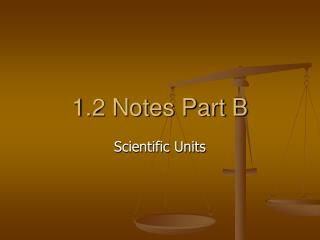DownloadDownload Presentation1.2 Notes Part B

# 1.2 Notes Part B

Download Presentation## 1.2 Notes Part B

- - - - - - - - - - - - - - - - - - - - - - - - - - - E N D - - - - - - - - - - - - - - - - - - - - - - - - - - -
##### Presentation Transcript

1. 1.2 Notes Part B Scientific Units

2. Unit Objectives • Use appropriate SI units for length, mass, time, temperature, quantity, area, volume and density. (ACT Quality Core I.A.2.b)

3. SI Units • Le Système International d’Unités (SI) • International System of Units in USA • A modern version of the metric system based on a decimal system • Understood throughout the world • Each type of measurement has a base unit • Multiples of 10

4. Length • Distance between 2 points • SI base unit = meter (m). • Metric rulers and meter sticks are used to measure length

5. Mass • The quantity of matter in an object • SI Base Unit = gram (g) • NOT the same as weight • Weight = Newton (N) = measure of gravitational force on an object

6. Volume • The amount of space occupied by an object • Can measure volume of a solid, liquid, or gas • SI base unit is the liter, L • A liter occupies the same volume as a cubic decimeter, dm3. • 1 L = 1 dm3 • (1 mL = 1 cm3)

7. Measuring Volume • FOR Solids • Measure length, width, and height • Multiply the three numbers and their units together (V = l x w x h) • Volume = expressed in cubic centimeters, cm3

8. SI Units • Time = second (s) = interval between 2 events • Temperature = Kelvin (K) = measure of the average kinetic energy of particles in an object • Kelvin = °C +273 • °C = Kelvin - 273 • Example: 20 °C + 273 = 293 K

9. Time • Time is the interval between two events. • Or the time it takes for something to happen • SI unit for time = second(s)

10. Temperature • Measure of how hot or how cold something is. • For most scientific work, temperature is measured on the Celsius (C) scale. • On this scale, the freezing point of water is 0C, and the boiling point of water is 100C. • Between these points, the scale is divided into 100 equal divisions. Each one represents 1C.

11. Temperature • SI Unit = Kelvin (K). • Absolute Zero (0K) = coldest possible temperature • Absolute zero is equal to -273C which is 273 below the freezing point of water.

12. Finding Kelvin • Kelvin temperature can be found by adding 273 to the Celsius reading. • On the Kelvin scale, water freezes at 273 K and boils at 373 K. • To find Kelvin simply add 273 to the °C • K = °C +273 • To find Celsius, subtract 273 from the K • °C = Kelvin - 273

13. Temperature • These three thermometers illustrate the scales of temperature between the freezing and boiling points of water.

14. Density • Density is the mass per unit volume of a material • You find density by dividing an object’s mass by the object’s volume

15. Density = Derived Unit • SI Unit = g/cm3 • A combination of SI units. • A unit obtained by combining different SI units or multiply an IS unit by itself = a derived unit.

16. SI Units • Length = meters • Mass = grams • Time = seconds • Temperature = Kelvin • Volume = Liters, cubic centimeters • Density = g/mL, g/cm3

17. In-Class Assignment/Homework • Page 879 #1-4, 6-10 Math Problems • 1 m = 10 dm =100 cm = 1000 mm • 1 dm = 10 cm = 100 mm • 1 cm = 10 mm • 1 L = 1 dm3 • 1 mL = 1 cm3 • 1.2 reinforcement WKT